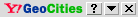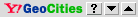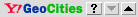undefinedundefined More...undefined [Close]

A Note: Why Modern Mathematicians Can't Understand Archytas

by Jonathan Tennenbaum

"As for me, I cherish mathematics only because I find there the traces of the Art of Invention in general, and it seems to me I have discovered, in the end, that Descartes himself did not yet penetrate into the mystery of this great science. I remember he once stated, that the excellence of his method, which appears only probable in terms of his physics, is proven in his geometry. But I must say, that it is precisely in Descartes' geometry that I recognized the principle imperfection of his method... I claim that there is an entirely different method of geometrical analysis, than that of Vieta and Descartes (i.e. algebra), who did not go far enough, because the most important problems do not depend at all upon the equations, to which Descartes reduces his geometry." (Leibniz, Letter to Princess Elisabeth, late 1678)

For example: the catenary, which requires {physical substance} for its generation, could not exist in the world of Descartes, Lagrange and Euler!

Looking through recent, standard presentations of Archytas' famous construction for doubling the cube, demonstrates how far modern mathematics has fallen below the level of thinking that prevailed in Plato's circles over 2300 years ago! Typical is a discussion of the doubling of the cube, on a webpage authored by J.J. O'Connor and E.F. Robertson (footnote 1). Although the text includes some interesting quotes and references, when the authors get to Archytas' actual construction, they shamelessly revert to the school-boy routine of "using coordinate geometry to check that Archytas is correct". Imposing a Cartesian coordinate system, they write down algebraic equations in x,y,z for each of the three intersecting surfaces (cone, cylinder and torus), and combine the equations to show that the desired proportionalities "somehow come out". Magic! Readers, foolish enough to engage in this meaningless exercise, will not only have learned less than nothing about Archytas' actual discovery; worse, the cognitive processes of minds will have been "turned off" altogether.

The stunning sophistication of Archytas' synthetic-geometrical approach, when viewed in terms of standard accounts of ancient Greek mathematics, suffices to demonstrate, that those standard accounts are grossly inadequate, and that the actual physical conceptions underlying his work have been suppressed. In fact, most of the crucial original documents of Greek science have been lost or destroyed, while the living continuity of Greek science was broken off through the "dark age" imposed under the Roman Empire. As an included result of that process, the surviving version of Euclid's famous "Elements" -- a compendium whose axiomatic-deductive mode of presentation buries the essential ideas and historical process of development of Greek science -- subsequently became, or was made into, the nearly exclusive source for classical Greek geometry, as well as the model for elementary mathematics education for many many centuries. Among other things, Euclid's "Elements" obfuscated the natural ordering of development, even in visual geometry, by beginning with {plane geometry} and the supposedly self-evident concepts of "point" and "straight line" as irreducible entities, proceeding only in the final chapters to the constructions of so-called solid geometry. Whereas, in the first and most "elementary" visual geometry is not "flat" {plane} geometry at all, but rather {spherical} geometry -- the form of geometry associated with astronomy as Man's oldest science.

These and related circumstances, explain why the greatest scientific thinkers, from the Renaissance through to Kepler and Leibniz, directed much of their efforts to reconstituting the actual method and "soul" of classical Greek mathematics, which could at best only be read "between the lines" of Euclid and certain other, mostly fragmentary surviving texts, and for which the surviving dialogs of Plato were the single most important source.

Crucial to this process, was the Renaissance revival of the isoperimetric principle, of circular and spherical geometry, and the significance of the five regular solids. Exemplary, in one respect, was the way Pacioli and Leonardo Da Vinci's "Divina Proportione" in effect "turned Euclid on his head" by emphasizing the primacy of Euclid's famous Thirteenth Book. Kepler carried the polemic further, developing a first approximation to a true physical geometry from the standpoint of the crucial evidence of the regular solids. This led directly into Fermat's, Pascal's and Leibniz's reworking of such items as Apollonius' Treatise on conic sections, in the context of a growing focus on the conception of higher-order, multiply-connected manifolds, which evidently lay at the center of the discussion among Plato's scientific collaborators. Thus, there is a direct line from Archytas and Apollonius, into the work of Gauss and Riemann.

From this standpoint I propose, for those eager to dig into the matter in some detail, the following observations on Archytas' construction for the doubling of the cube. Although my observations are somewhat technical, and do not aim at a full representation of his discovery, they should help put us on a fruitful track, repairing some of the damage caused by modern misrepresentations.

I assume, in the following, that the reader already has some familiarity with Archytas' construction, from previous discussions by Bruce Director and others, including the relationship between doubling the cube, and the general problem of constructing two "mean proportionals" between given lengths a and b. (footnote 2)

The Geometry of Physical Events

Note, firstly, that by deriving the solution by means of an intersection of a torus, cone and cylinder Archytas situates the problem explicitly in the domain of multiply-connected, "polyphonic" circular action. Observe the emphasis on {verbal action}, reflected in the classical account of Archytas' construction, by the geometer Eudemus:

"Let the two given lines be OA [= a] and b; it is required to construct two mean proportionals between a and b. Draw the circle OBA having OA as diameter where OA is the greater [of the two]; and inscribe OB [as a chord on the circle] of length b, and prolong it to meet at C the tangent to the circle at A. ... Imagine a half-cylinder which rises perpendicularly on the semicircle OBA, and that on OA is raised a perpendicular semicircle standing on the [base] of the half-cylinder. When this semicircle is moved from A to B, the extremity O of the diameter remaining fixed, it will cut the cylindrical surface in making its movement and will trace on it a certain bold curve. [The latter motion generates a section of a torus--JT.] Then, if OA remains fixed, and if the triangle OCA pivots about OA with a movement opposite to that of the semicircle, it will produce a conical surface by means of the line OC, which, in the course of its movement, will meet the curve drawn on the cylinder at a particular point P...."

What a contrast between the indicated polyphonic conception of geometry, and today's mind-deadening "set theory"! In Archytas' construction, P arise not as an intersection of static "point sets", but as the locus of a physical {event}, whose process of generation involves three (or actually, six) simultaneous degrees of action. Achytas designs the process in such a way, that the event, so generated, will possess exactly the required "projective" relationships. In particular, the required "two mean proportionals" are OQ and OP, where Q is the projection of the point P, constructed as above, onto the plane of the original circle OBA.

But, before attempting to derive Archytas' construction by ourselves, let us look at the simpler case of the relationship between the geometric mean and circular action. This gives us a suitable jump-off point for tackling the problem solved by Archytas.

Harmonic Proportions and Circular Action

Circular rotation provides the simplest, characteristic case for the generation of harmonic proportions among what are ostensibly scalar magnitudes (line segments, for example), as a "projected" result of higher-order action.

Construct a circle with a given diameter OA. A point P, moving along the circle between O and A, gives rise to an array of invariant harmonic proportions, in the following manner.

Connecting P with the endpoints of the diameter, O and A, produces a triangle OPA, whose shape changes with P's position, but whose angle at P is always a right angle. Now project P perpendicularly to the line OA, calling the point of projection "Q". Evidently, the triangle OPQ is also a right triangle (right angle at Q), and it shares a common angle at O with the original right triangle OPA. The two triangles are thus constantly similar, throughout P's motion, and the corresponding ratios of the sides will be equal. In particular, OQ:OP = OP:OA. This amounts to saying, that the length OP is the {geometric mean} between OQ and OA. By inverting the order of construction, we can generate the geometric mean of any two given lengths OQ and OA, using the circle. Just project Q onto the circumference of the circle, to get the point P.

The geometric mean was also known in ancient Greek times as a "single mean between two extremes". Doubling a {square}, requires constructing such a single (geometrical) mean between 1 and 2. To double a cube, however, we need {two} means between 1 and 2, or in other words a series of simultaneous proportions of the form: OB:OQ = OQ:OP = OP:OA where OB and OA have lengths 1 and 2, respectively.

Now, since the above construction already generates "half" the required proportion, namely OQ:OP = OP:OA, the following strategy immediately suggests itself:

"Introduce a {second} degree of rotation, generating the "other half" of the double proportion, namely: OB:OQ = OQ:OP.

"Thus, all we need to do is to somehow combine the two circular actions, in order to generate an {event}, at which both conditions are realized {simultaneously}; this will give us the required double mean: OB:OQ = OQ:OP and at the same time OQ:OP = OP:OA."

Carrying out this strategy does lead to a construction for the double mean, albeit one that is open to certain criticisms. I present it briefly, because it already points in the direction of multiply-connected action.

In fact, to get the proportion OB:OQ = OQ:OP in the indicated manner, we need to construct a {second} circle, of diameter OP, such that (i) the point Q (P's projection on the diameter of the first circle) also lies on the second circle, and (ii) Q projects to a point B on the second circle's diameter OP, such that the distance OB has the required length 1.

A bit of geometry, shows that requirement (i) is actually satisfied for {all} positions of P on the first circle; requirement (ii), however, is fulfilled only for {one} position of P (and its symmetrical image). How might we generate that locus as a constructible {event}?

Simple, in principle! Imagine, that for each position of P, as P moves along the first circle, a corresponding circle is constructed around OP as diameter. This process produces a continuous {family} of circles, whose diameters OP are changing angle and length as P moves (footnote 3). Now mark off, on each diameter OP, a point B', such that OB' has length 1; and let Q' be the corresponding point on the circumference of the corresponding circle, so that B' is the projection of Q' onto OP. (Of the two possible choices for Q', choose the one lying inside the original, first circle.) The points Q', so determined, describe a {curve} inside the first circle. Looking at various positions of P, we can easily see that the curve has points on both sides of the first circle's diameter OA, and must therefore {cross} it at some point.

That crossing is the required event! At that moment, the points Q and Q' coincide, and both parts of the indicated double proportion hold simultaneously. OQ is the side of the cube, whose volume is twice that of the cube with unit length.

Now one might, with some justification, object, that no method was presented above, for how to actually {draw} the curve defined as the locus of points Q'. It is obviously not enough to simply demand: "Mark, on each one of the infinite family of circles, a corresponding point Q'". For, if we were to begin to mark circles and points one at a time, we would never have anything more than a discrete set, and would never arrive at a continuous curve. (footnote 4)

On the other hand, it is quite possible, with a bit of ingenuity, to design a relatively simple {physical mechanism} that traces the required curve as a product of the motion of P along the circumference of the original circle. The resulting method is akin to the tactic of Nichomedes, who used a mechanically-generated curve called the {conchoid}, to double a cube.

Back to Archytas

From this standpoint we may now better appreciate the singular breakthrough of Archytas, who went far beyond the above "ad hoc" methods, to discover a higher-order approach to the problem which anticipates Gauss' 1799 grounding of the complex domain, by over two millenia!

By applying a new degree of rotation to the first circle, to generate a {torus}, Archytas takes us, by implication, into an {entirely new universe}. Instead of trying to build the solution "from the bottom up", as before, we can now proceed more "from the top down".

The torus in question, is obtained by rotating the original circle first into the vertical plane (with O fixed) and then rotating the resulting circle around the vertical axis through O. For any point P on the torus, the vertical cross section through the axis of the torus, is a circle of diameter OA (of length 2); if Q denotes P's projection onto the horizontal diameter of that circle, the proportion OQ:OP = OP:OA will hold -- now as an invariant relationship for the {entire surface} of the torus.

Now it is easy to introduce additional degrees of circular action, generating additional harmonic relations. If, for example, we cut the torus by a vertical cylinder which contains the vertical axis (and the point O), then any point P, lying on the intersection of the two surfaces, automatically belongs to {two} circles: (i) the vertical section of the torus through P, as already described, giving rise to the relation OQ:OP = OP:OA, and (ii) the vertical section of the cylinder through P. In the vertical projection of that circular section onto the horizontal plane, P projects to the already-mentioned point Q. Lying on the projected circle, Q generates a {second} set of harmonic relations, of the form: OR:OQ = OQ:OD, where OD is the diameter of the cylinder (and the projected circle) and R is Q's "lateral" projection onto the diameter OD. So far the length of OD (the diameter of the vertical cylinder) is variable; we can chose any value we want. Archytas chooses it to be equal to OA, in which case the projected circle coincides with the original one. But in principle many other choices would be possible.

In any case, we have room for introducing still a {third} principle. Remember, that our immediate object is to generate an event at which, in addition to the invariant relation OQ:OP = OP:OA, a second relation OB:OQ = OQ:OP, or 1:OQ = OQ:OP (since OB = 1) holds. Compare this with the relation we just generated using the cylinder: OR:OQ = OQ:OA.

A bit of reflection, shows that the latter relationship is equivalent to: 1:OQ = OQ:(OA x OR)

Thus, to get the relationship we are looking for, namely 1:OQ = OQ:OP, all we need to do, is generate an event, where OA x OR = OP. Since OA has length 2, this amounts to saying, that OR should be 1/2 the length of OP.

How are OP and OR related? Very simply, as one can see: R is the direct, perpendicular projection of P onto the axis OA, i.e. the point at which the vertical plane through P, drawn perpendicular to the axis OA, intersects that axis. Taken by itself (leaving aside the other constraints on P), the requirement that OR be equal to 1/2 OP, is equivalent to saying, that P lies on a certain {cone} with apex O and axis OA. The required cone can easily be constructed; this, indeed, is the preliminary step which Eudemus describes.

By this road -- admittedly a bit bumpy in places --, we arrive at Archytas' construction. This time not to verify it, but to derive it by ourselves. ----------------------------------

1. See www.history.mcs.standrews.ac.uk/history/HistTopics/ Doubling_the_cube [Try http://www-groups.dcs.st-and.ac.uk/~history/HistTopics/Doubling_the_cube.html]

2. Briefly: by "two mean proportionals" are understood two magnitudes x and y, between given magnitudes a and b (a assumed larger than b) such that b:x = x:y = y:a. Doubling the cube corresponds to the special case a = 2 and b = 1. The first of the two mean proportionals, x, corresponds to the side of a cube having double the area of the unit cube. The second mean, y, corresponds to the area of a side of the new cube.)

3. Alert readers will note here the traces of {conical action}, which becomes more explicit in Archytas, and finally emerges in full clarity in Gauss' complex domain.

4. For related reasons, mathematics as generally conceived cannot represent true continuity, but at best describe certain {results} of continuous action. Only the direction of development of mathematics, set forth by Leibniz in his original conception of the calculus, and continued by Riemann, provides a pathway of development of mathematics in the direction of ever more adequately representing the reality of continuous action in the Universe. The case of the catenary is exemplary.